Courses
Courses for Kids
Free study material
Offline Centres
More

# Important Questions for CBSE Class 8 Maths Chapter 10 - Visualising Solid ShapesLast updated date: 28th Nov 2023
Total views: 565.8k
Views today: 11.65k

## CBSE Class 8 Maths Important Questions for Visualising Solid Shapes - Free PDF Download

CBSE Class 8 Maths Chapter 10 Visualising Solid Shapes is a crucial part of the syllabus of this subject that explains different regular and irregular solids and their features. The exercises have been set by following these concepts. After completely preparing this chapter, students will need the important questions designed by the subject experts of Vedantu to practice. These questions come with solutions for adding more convenience to the practice sessions.

Free PDF download of Important Questions with solutions for CBSE Class 8 Maths Chapter 10 - Visualising Solid Shapes prepared by expert Mathematics teachers from the latest edition of CBSE (NCERT) books is available on Vedantu. Register online for Maths tuition on Vedantu.com to score more marks in your examination.

Vedantu is a platform that provides free NCERT Solutions and other CBSE study materials for students. Students can register and get access to the best and most reliable source of study materials specially made by master teachers at Vedantu. Subjects like Science, Maths, and English will become easy to study if you have access to NCERT Solution for Class 8 Science, Maths solutions and solutions of other subjects.

## Study Important Questions for Class 8 Maths Chapter 10 – Visualising Solid Shapes

Very Short Answer Type Questions (1 Mark)

1. A three dimensional shape is $\_\_\_\_\_\_\_\_\_$object.

Ans: Solid

2. The most important part of a map is the $\_\_\_\_\_\_\_\_\_$

Ans: Scale

3. What are three views in a solid figure?

Ans: Front view, side view and top view

4. Name of the solid whose net diagram is given below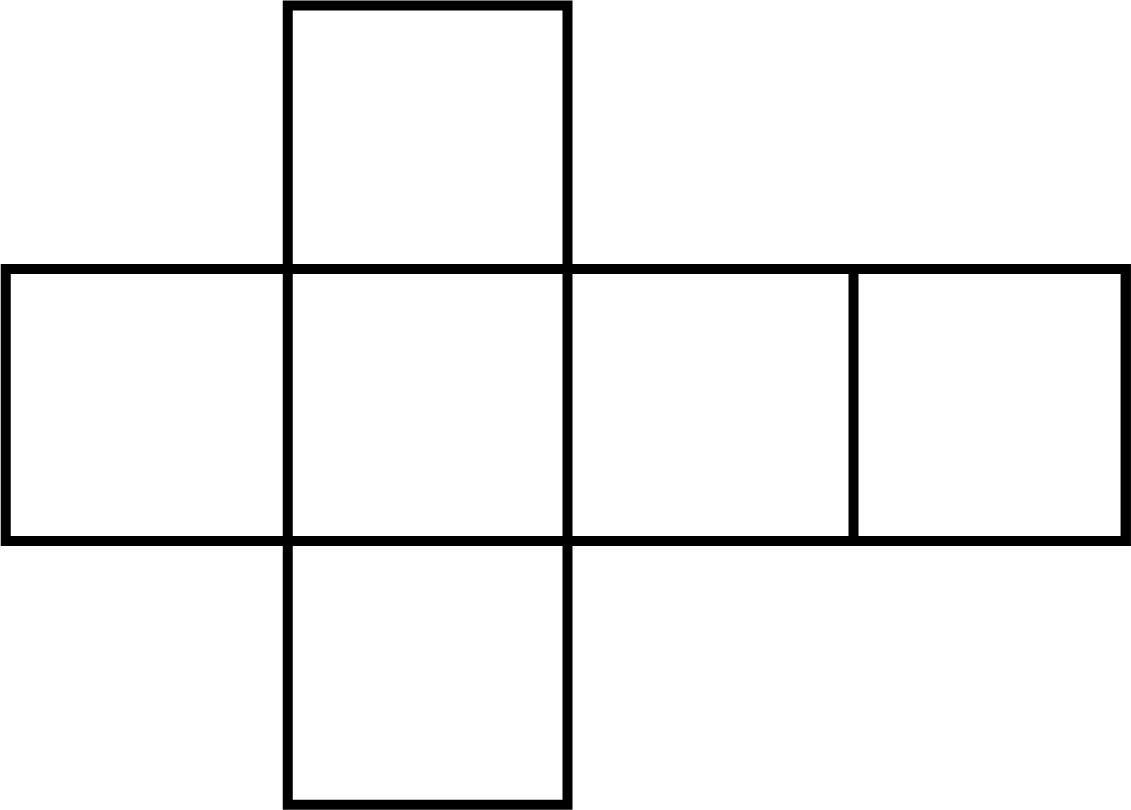(a) Pyramid

(b) Cone

(c) Cube

(d) Cuboid

Ans: (c) Cube

5. The number of triangular face's of a triangular prism is $\_\_\_\_\_\_\_\_\_$

(a) $1$

(b) $4$

(c) $2$

(d) $3$

Ans: (c) $2$

Short Answer Type Questions (2 – Marks)

6. What are regular polyhedrons?

Ans: A polyhedron is called regular if its faces are made up of regular polygons and the same number of faces meet a vertex.

7. Give two basic differences between a prism and a pyramid?

Ans: (a) Pyramid is a polyhedron in which the base is a polygon whereas a prism is a polyhedron in which the base and top are regular polygons.

(b) The lateral surface are parallelograms in a prism whereas in a pyramid the lateral surfaces are triangles.

8. Give two examples of $2\;{\text{d}}$ and $3\;{\text{d}}$ shapes each.

Ans: $2\;{\text{d}}$ shapes : circle, square.

$3\;{\text{d}}$ shapes = pyramid, cone.

9. How many number of squares are visible in top view and front view of the given figure.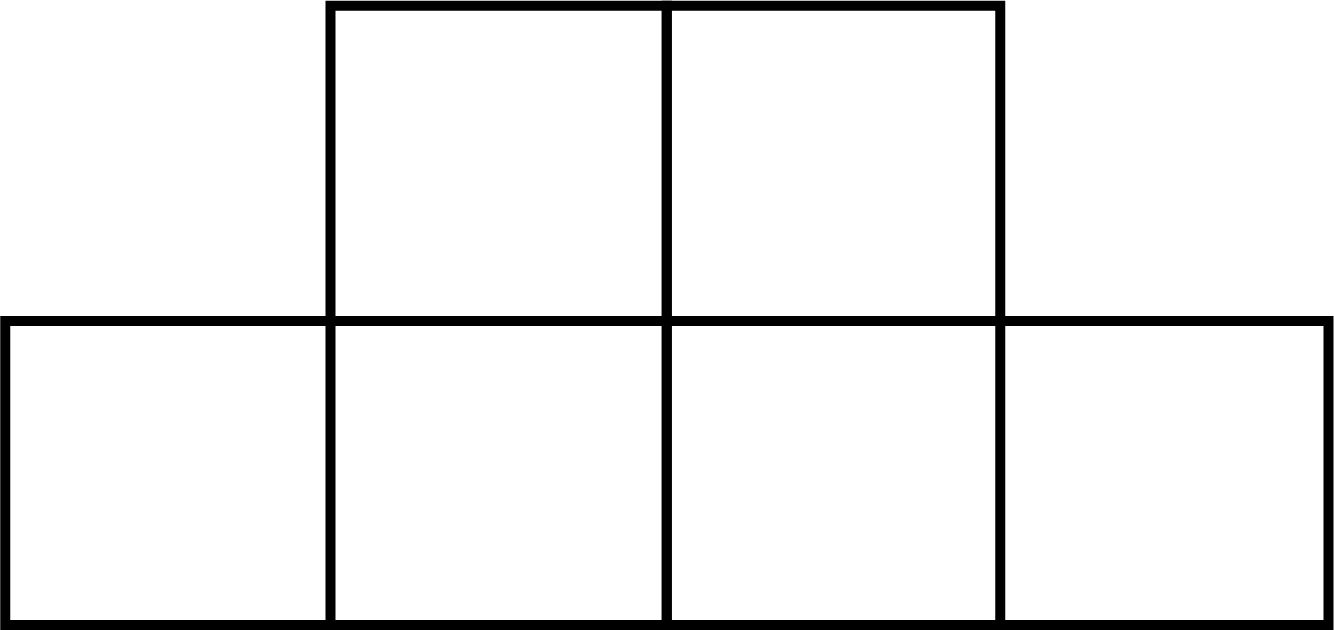Ans: Top view $= 6$ squares

Front view $= 4$ squares

10. In the given solid identify the top, front and side view.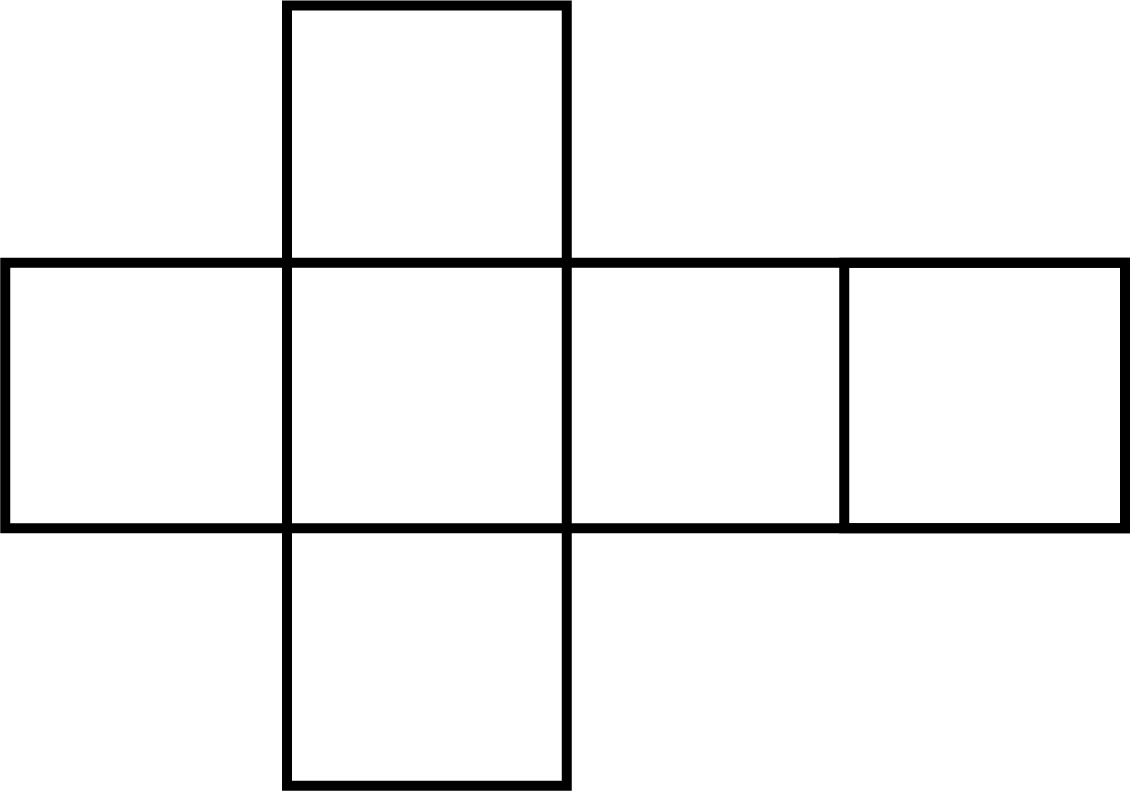(a)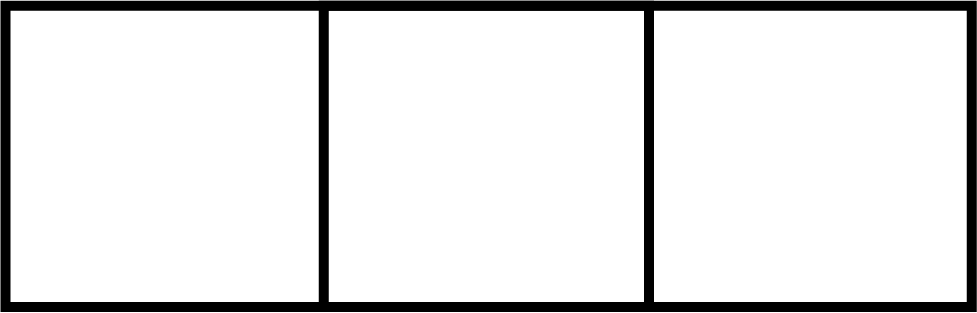(b)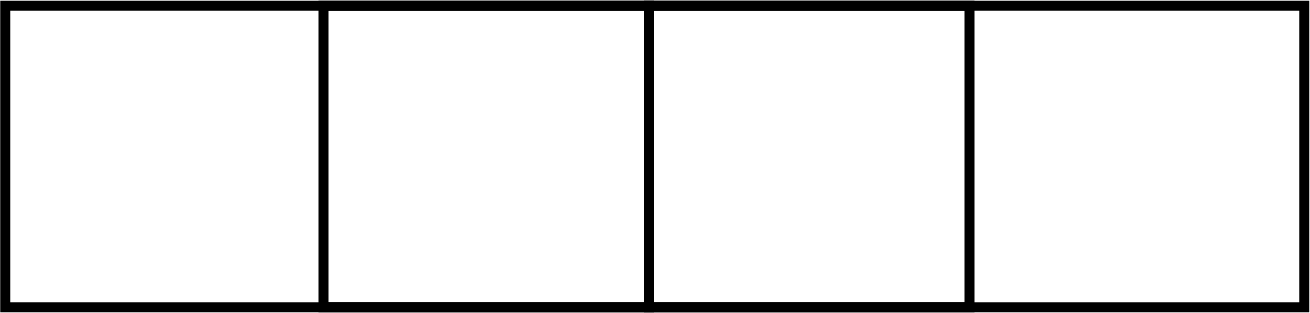(c)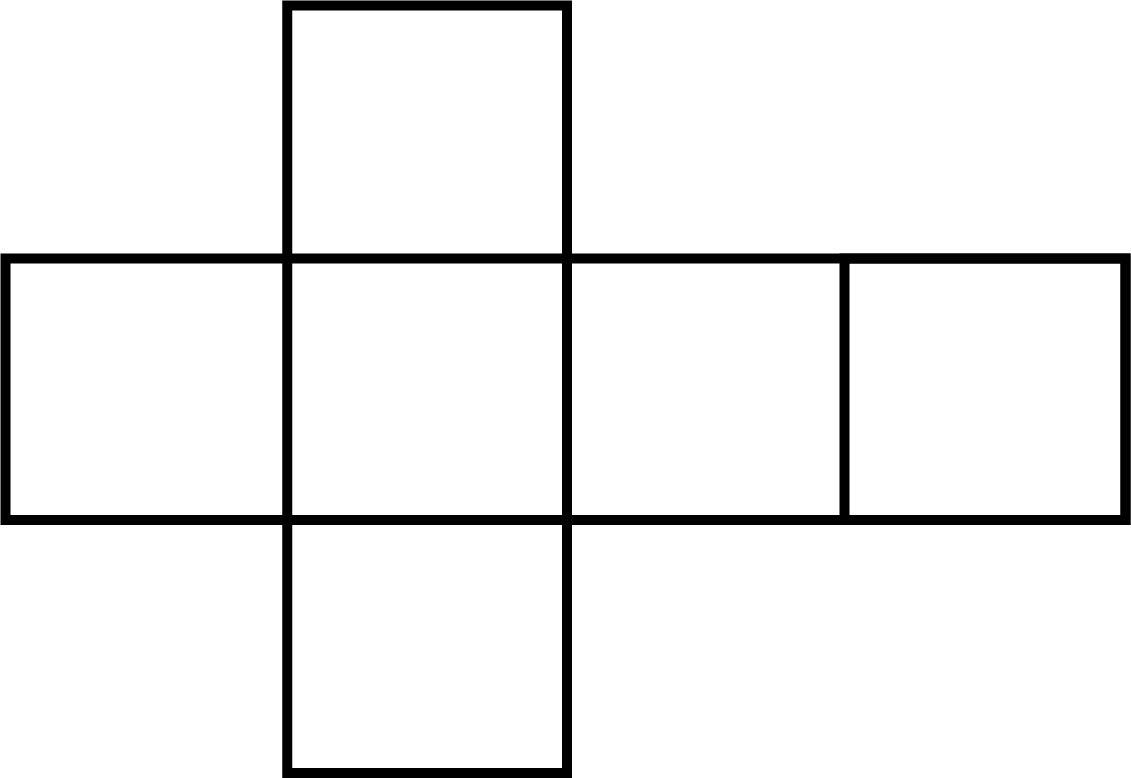Ans:

(a) side view

(b) front view

(c) top view

11. Give Euler's formula and explain each term in it.

Ans: In a polyhedron, the relationship between the number of faces $({\text{F}})$, vertices $({\text{V}})$, and edges $({\text{E}})$ is given by $F + V - {\text{E}} - 2$.

12. Write the number of faces, number of vertices and number of edges of a prism with rectangular base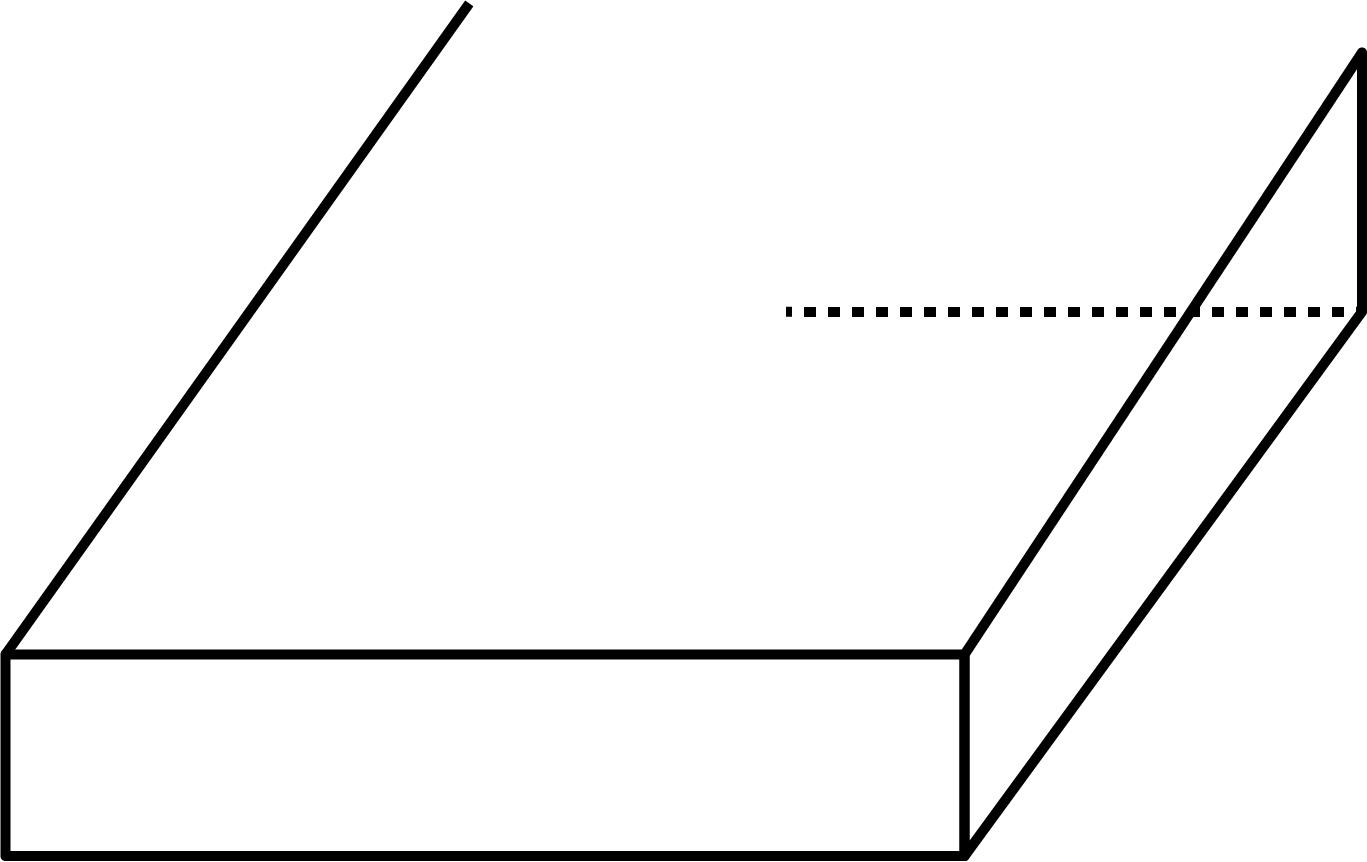Ans: No. of faces $= 6$

No. of vertices $= 8$

No. of Edges $= 12$

13. Write the number of faces, number of vertices and number of edges of a pyramid with hexagonal base.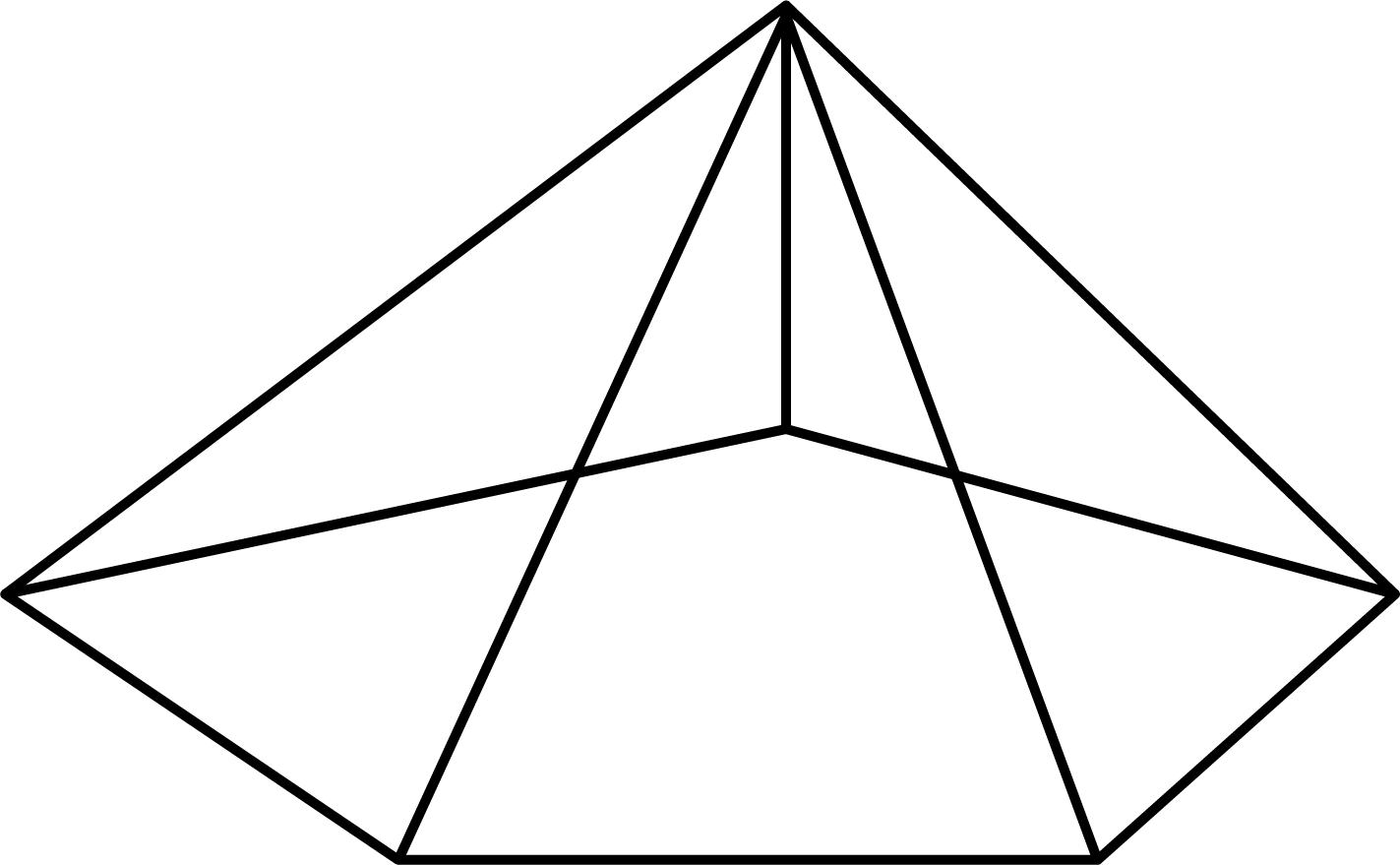Ans:

No. of faces $= 7$

No. of vertices $= 7$

No. of edges $= 12$

14. In a polyhedron there are $8$ vertices and $12$ edges. Find its number of faces.

Ans:

${\text{F}} + {\text{V}} - {\text{E}} = 2$

$F + 8 - 12 = 2$

$F = 2 + 12 - 8$

$F = 6$

15. A pyramid with square base has $5$ faces and $8$ edges. By Euler's formula, find the vertices of the pyramid.

Ans: For a pyramid,

$F = 5,{\text{ }}E = 8,{\text{ }}V = ?$

Using Euler's formula,

$F + V - E = 2$

$5 + V - 8 = 2$

$V = 2 + 8 - 5$

$V = 5$

Short Answer Type Questions (3 – Marks)

16. Draw the front view, side view and top view of the given objects.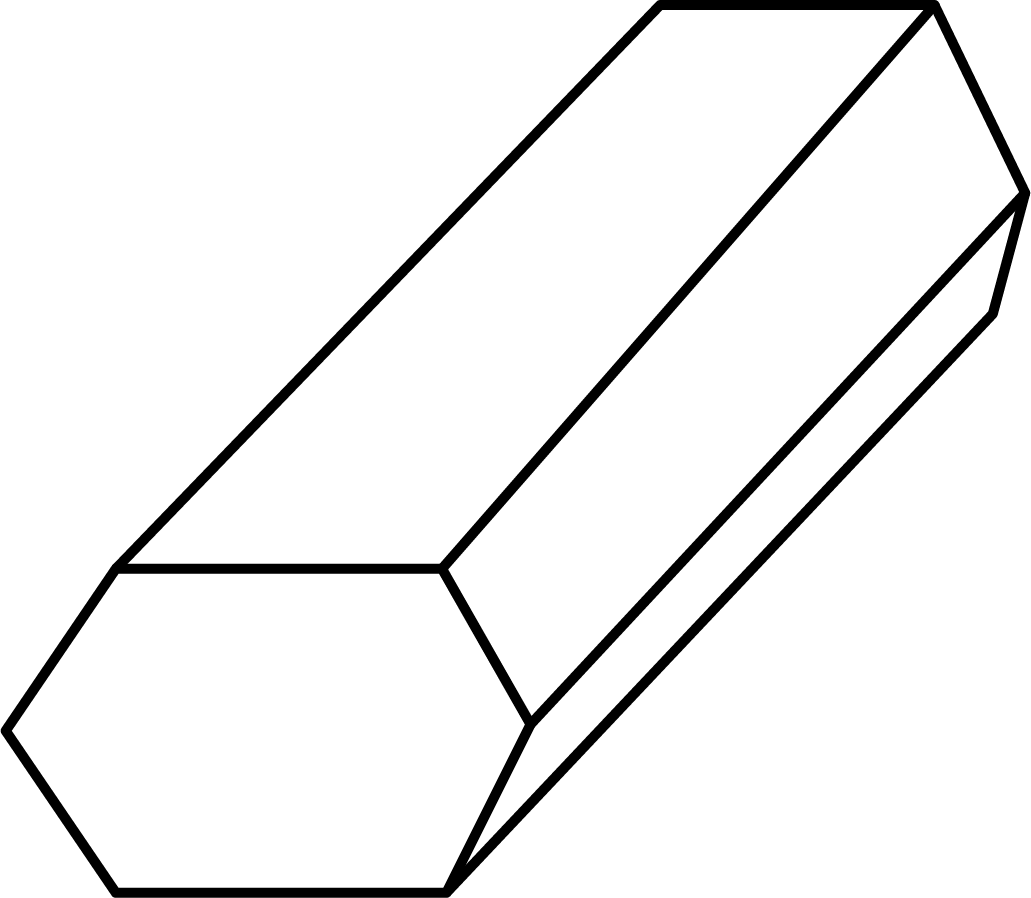Ans:

(a) Front view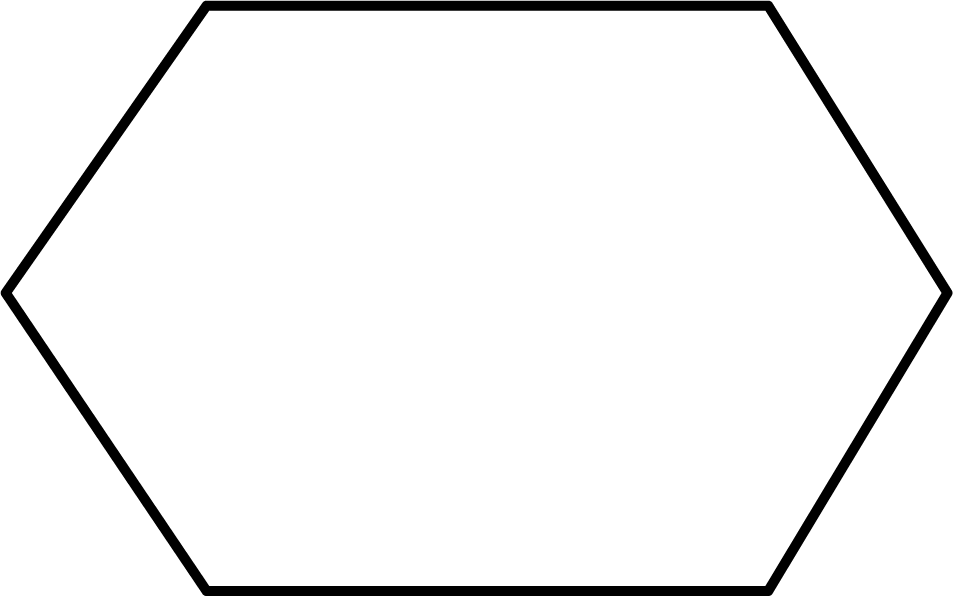(b) Top view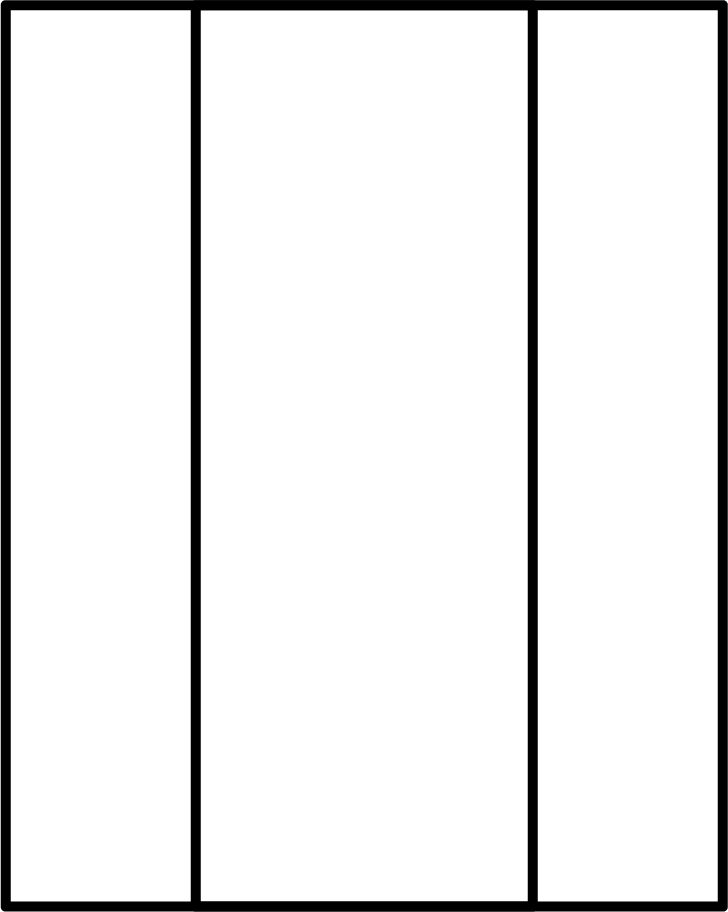(c) Side view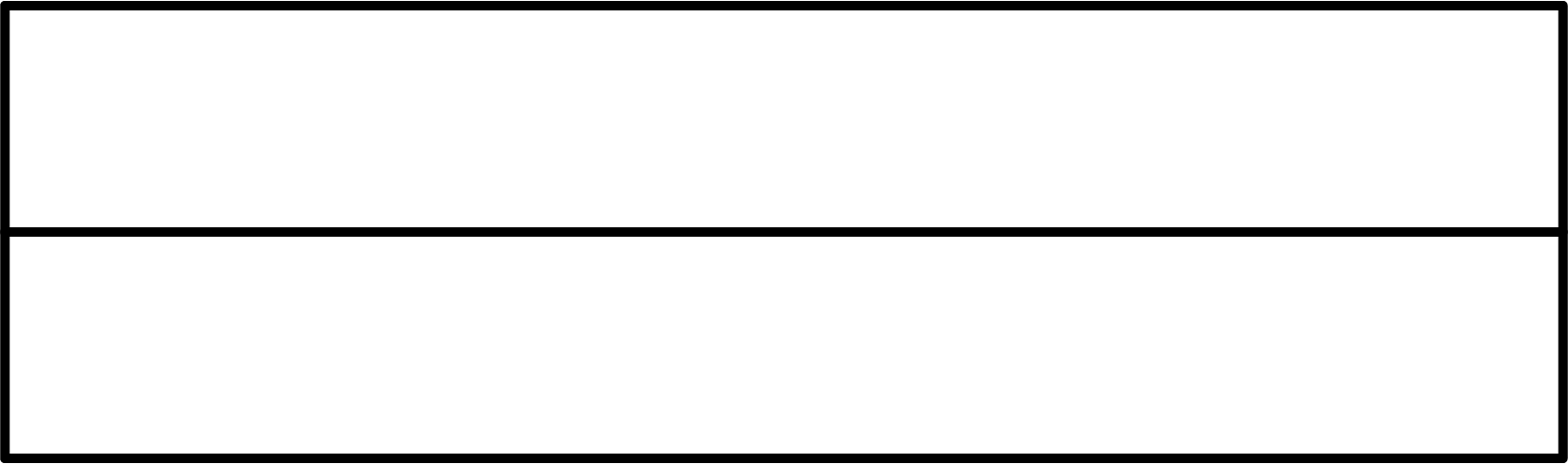17. Can a polyhedron have $10$ faces, $20$ edges and $15$ vertices? Justify your answer.

Ans: $F = 10,{\text{ }}E = 20$ and $V = 15$

Using Euler's formula,

$F + V - E = 2$

L.H.S:

$F + V - E = 10 + 15 - 20$

$F + V - E = 5$

$\therefore F + V - E \ne 2$

This polyhedron is not possible.

18. Match the following pictures with their shapes.

Ans:

 Picture (object) Shape (1)(a) A cone taken out of a cylinder (2)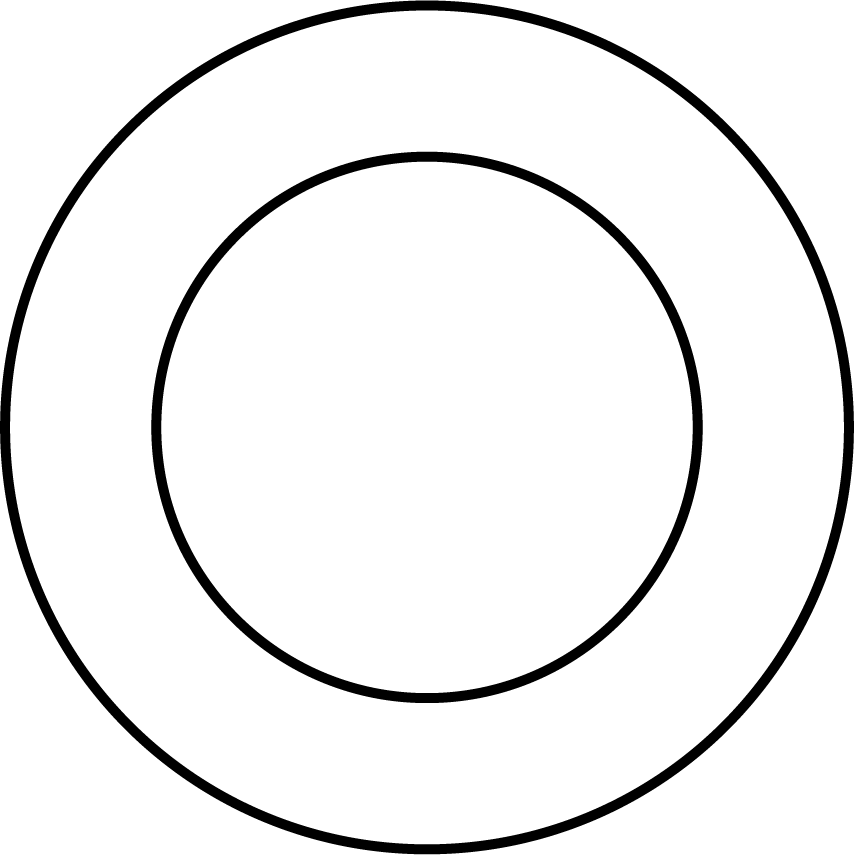(b) Two rectangular cross paths inside a rectangular park (3)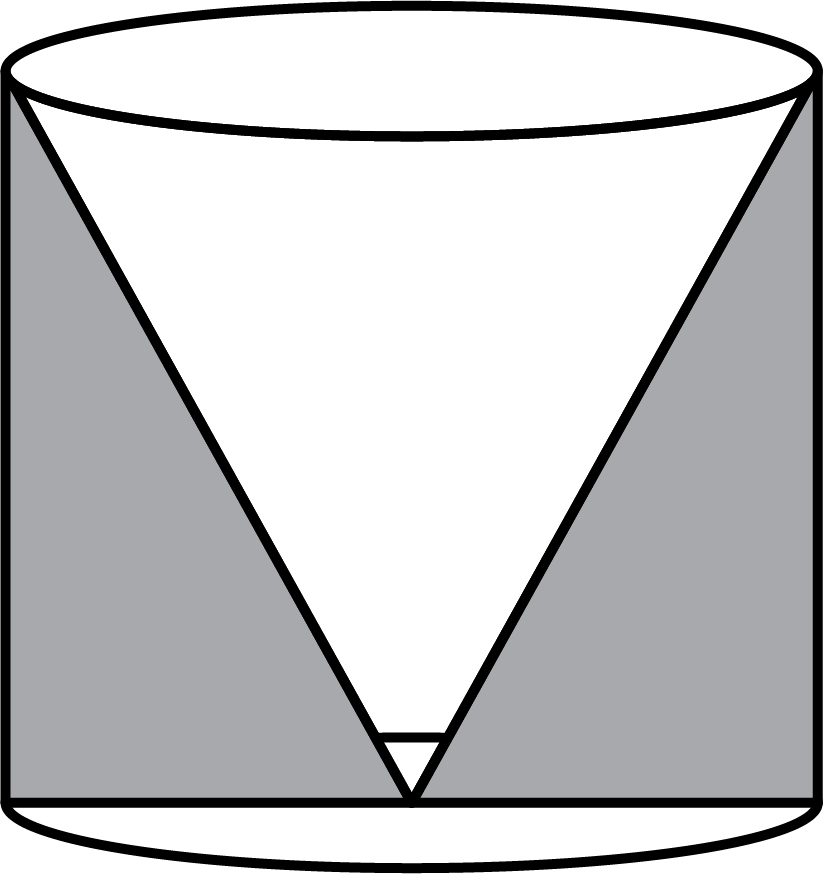(c) A circular path around a circular ground

Ans: $1 - b,{\text{ }}2 - c,{\text{ }}3 - a$

19. Define

(a) Face

(b) Edge

(c) Vertex

Ans: (a) Face$- {\text{A}}$$3\;{\text{d}}$ solid object is made up of flat surfaces or polygonal regions known as faces.

(b) Edge: In $3\;{\text{d}}$ solid objects, the faces meet at edges.

(c) Vertex: The point where all edges meet is known as a vertex.

20. By using Euler's formula find the unknown?

(a) vertices$= 12$, Faces$= 4$, Edges $- ?$

(b) Faces$= 5$, Edges$= 8$, Vertices $= ?$

(c) Edges$= 2$, Vertices$= 3$, Faces $= ?$

Ans: Using Euler 's formula:

$F + V - E = 2$

(a) $4 + 12 - E = 2$

$E = 16 - 2 = 14$

(b) $5 + V - 8 = 2$

$V = 2 + 8 - 5 = 5$

(c) $F + 3 - 2 = 2$

${\text{F = }}2 + 2 - 3 = 1$

Long Answer Type Questions (4 – Marks)

21. Write an examples for tetrahedron and write the number of triangular faces, vertices and edges. Show it verifies Euler’s formula.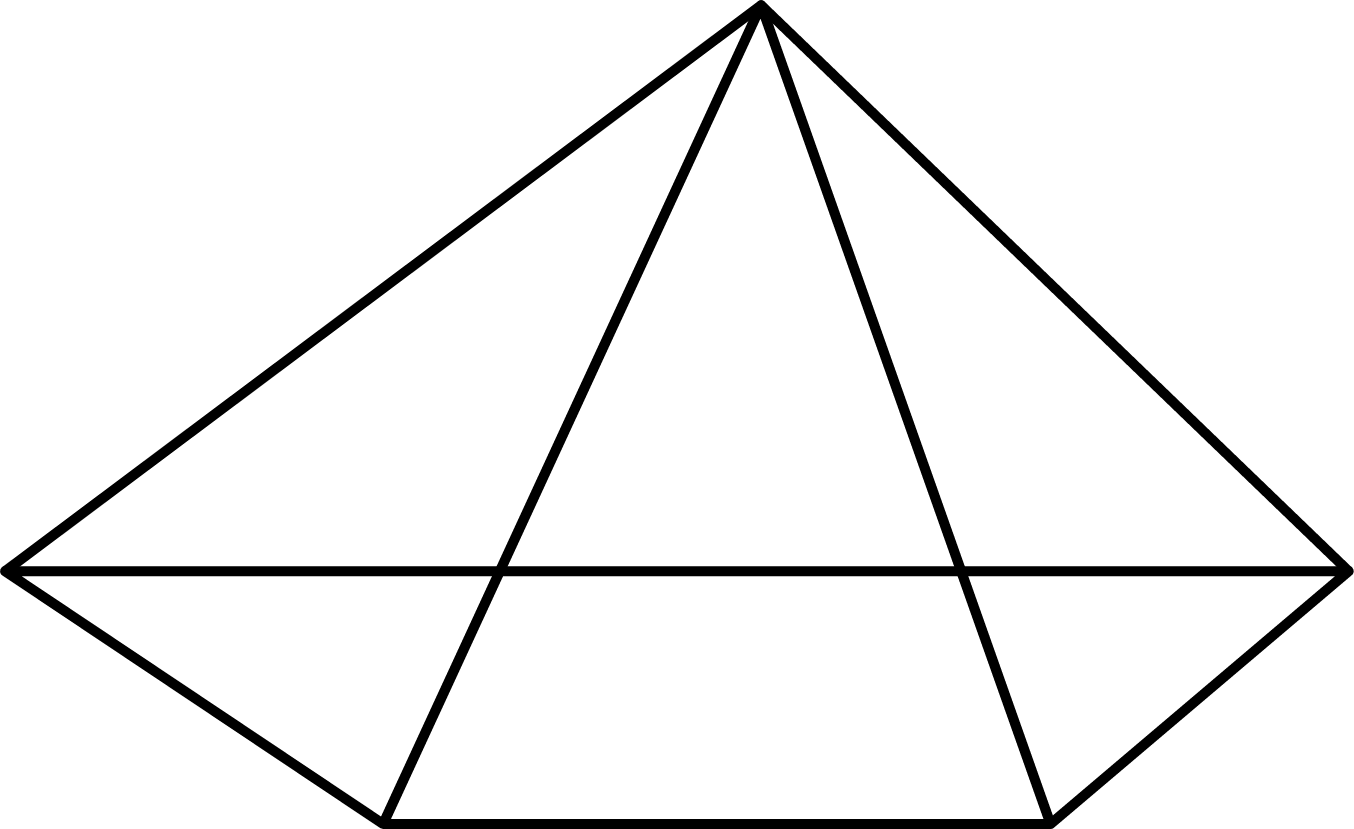Ans: Example for tetrahedron$=$Tent

Number of triangular faces $= 4$

Number of Vertices $= 4$

Number of Edges $= 6$

Euler's formula:

$F + V - E = 2$

$4 + 4 - 6 = 2$

$2 = 2$

Hence verified.

22. Write an example for Hexahedron with number of faces, vertices and edges. Show it verifies Euler's formula.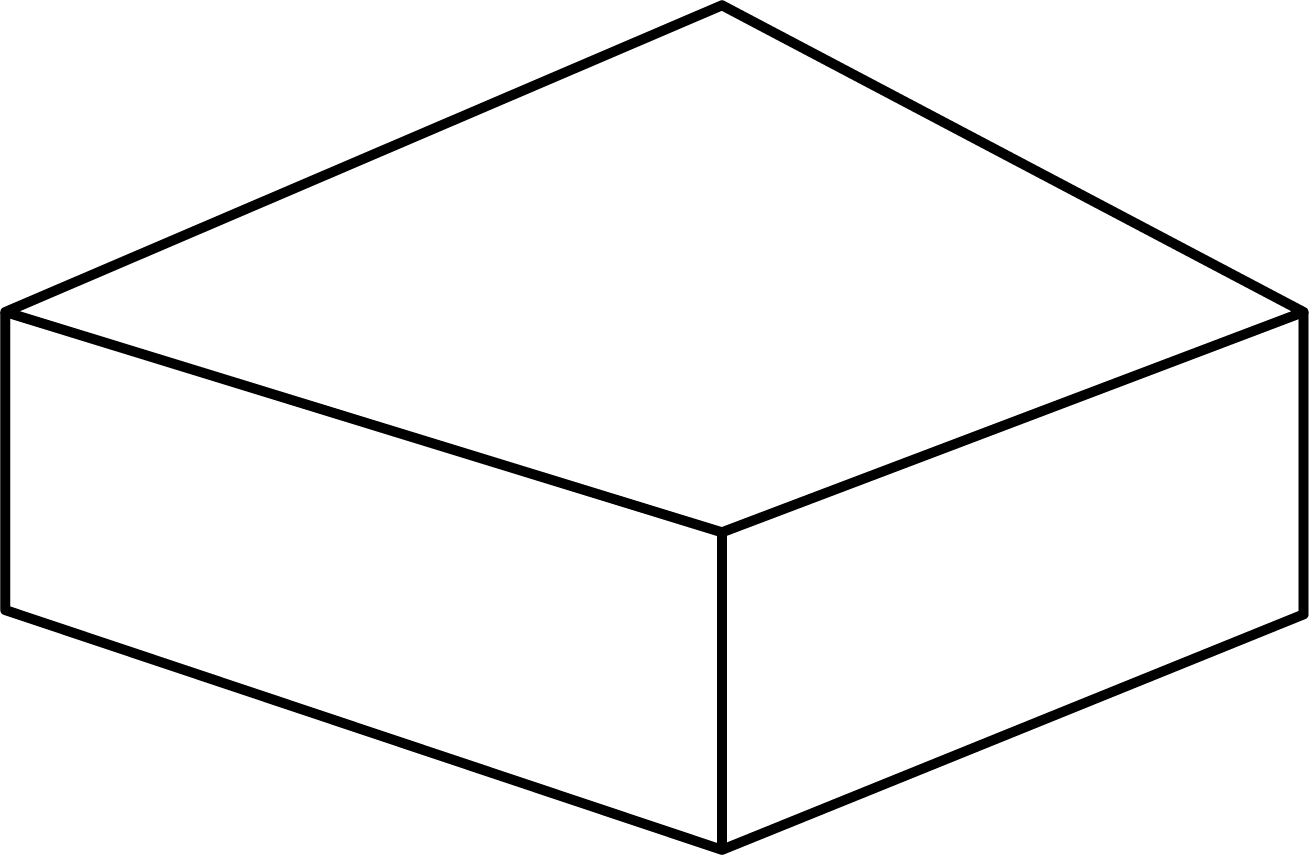Ans: Example for Hexahedron$-$Cube (Die)

Number of faces $= 6$

Number of Vertices $= 8$

Number of Edges $= 12$

Euler’s formula:

$F + V - E = 2$

$6 + 8 - 12 = 2$

$14 - 12 = 2$

$2 = 2$

Hence verified.

23. Write a prism with square base and a pyramid with rectangular base. Write the number of edges in each case.

Ans: (a) A prism having a square base has $12$ edges.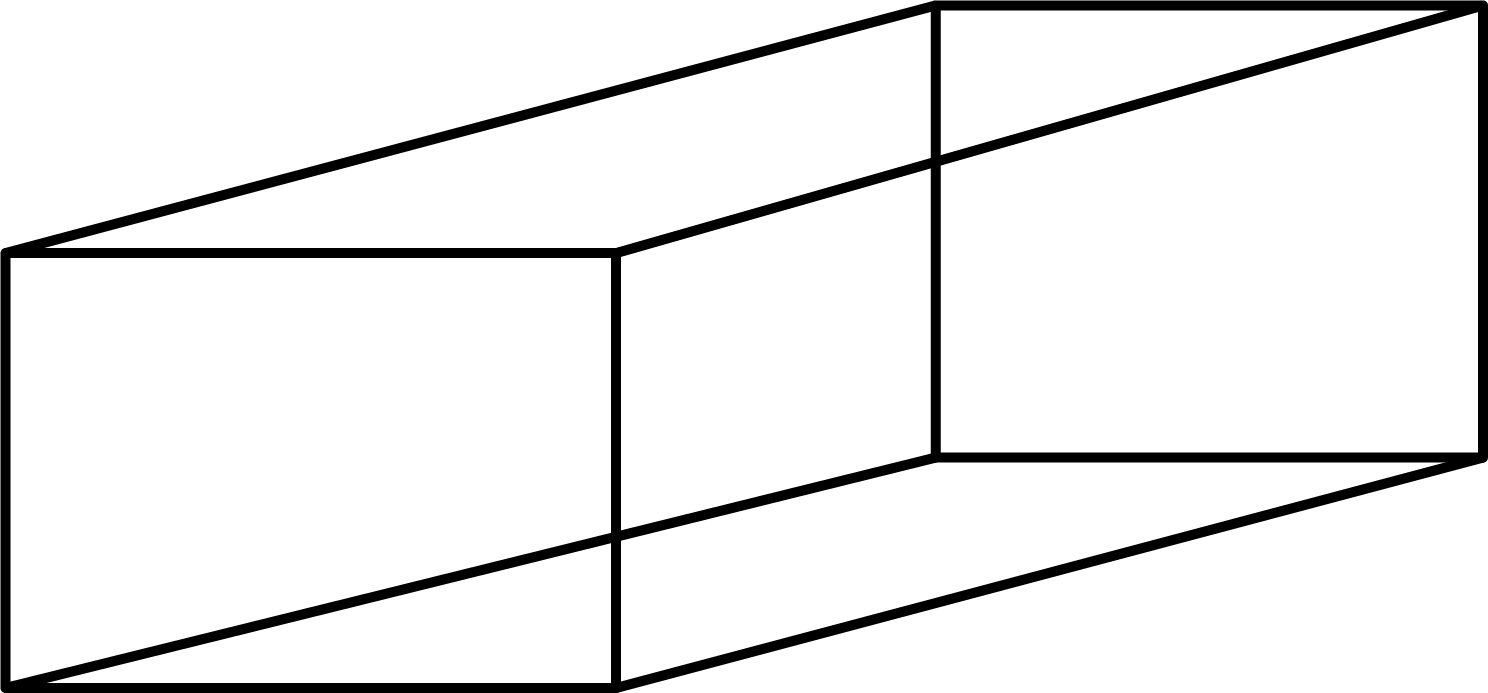(b) A pyramid with rectangular base has $8$ edges.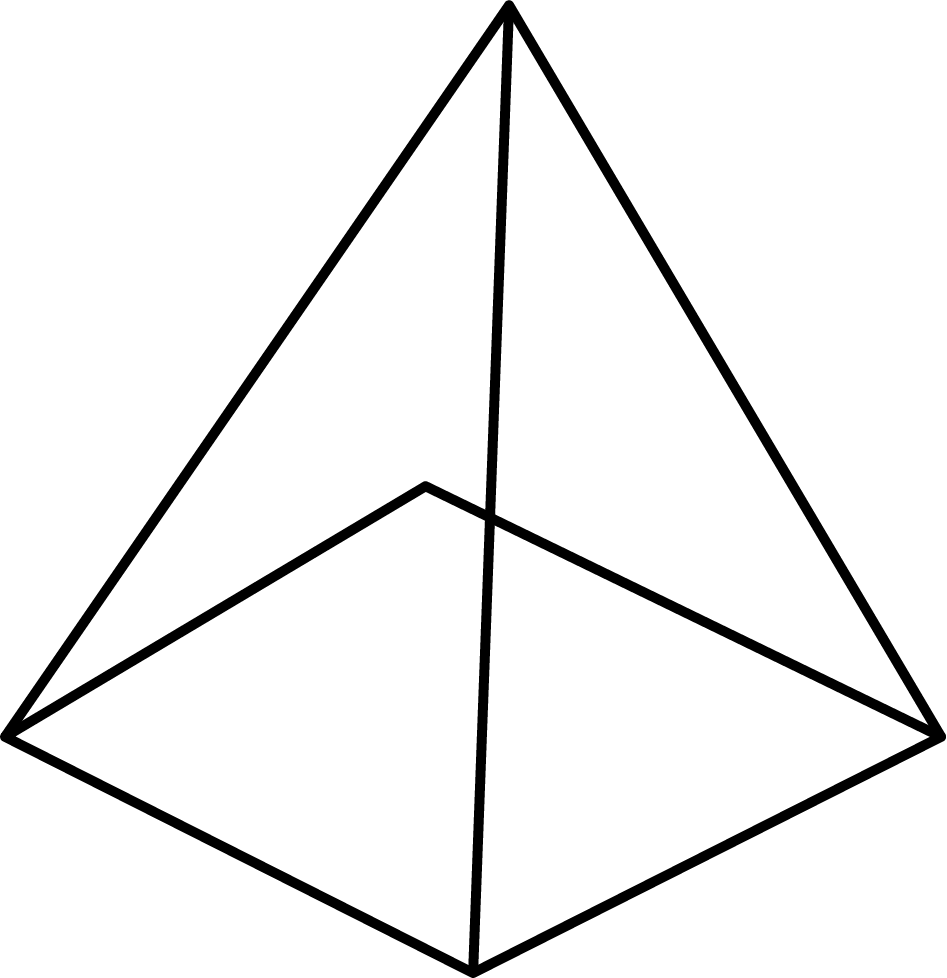24. Match the net’s with appropriate solids.

 (a)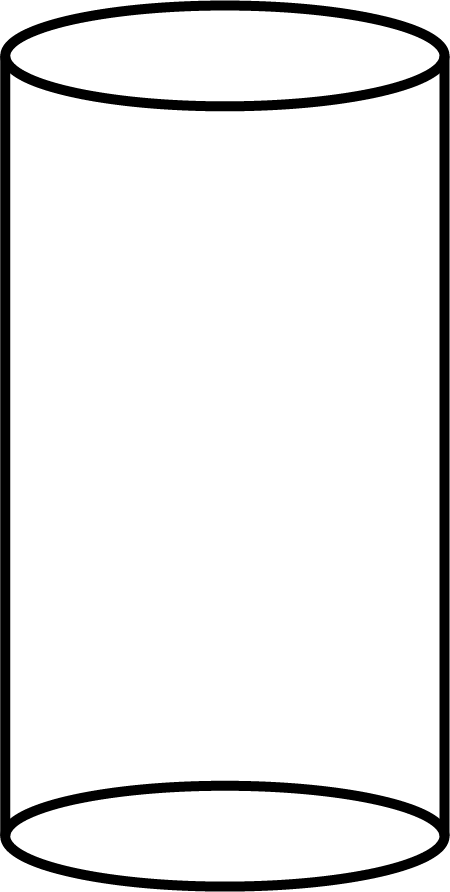(i)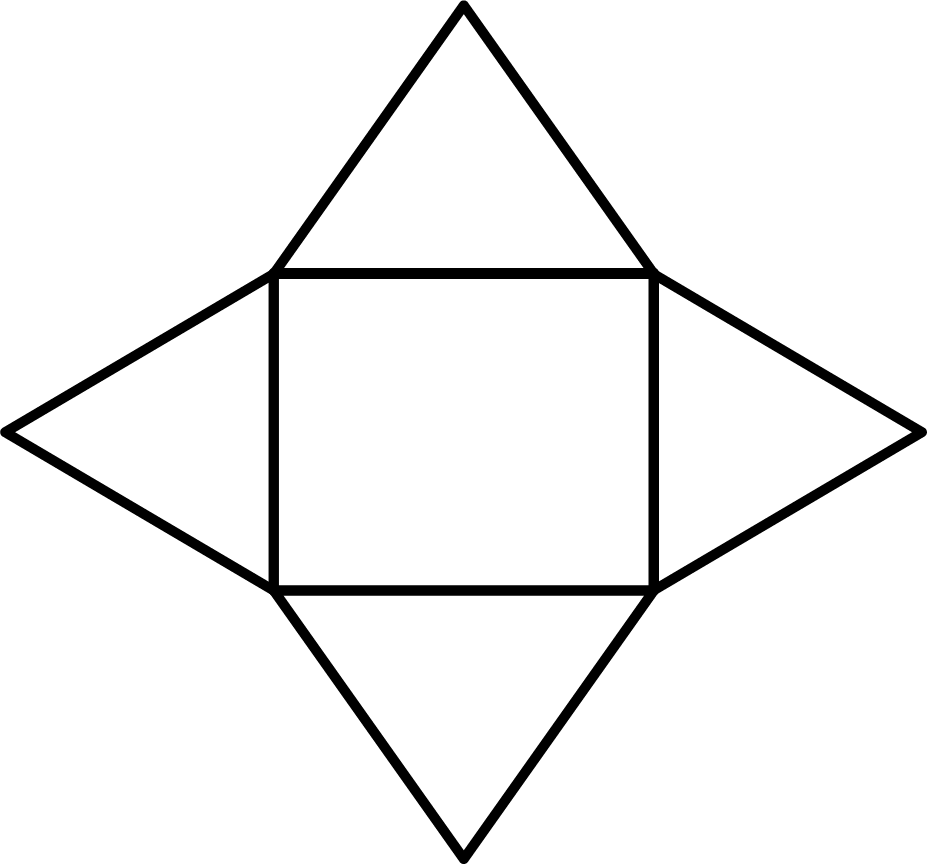(b)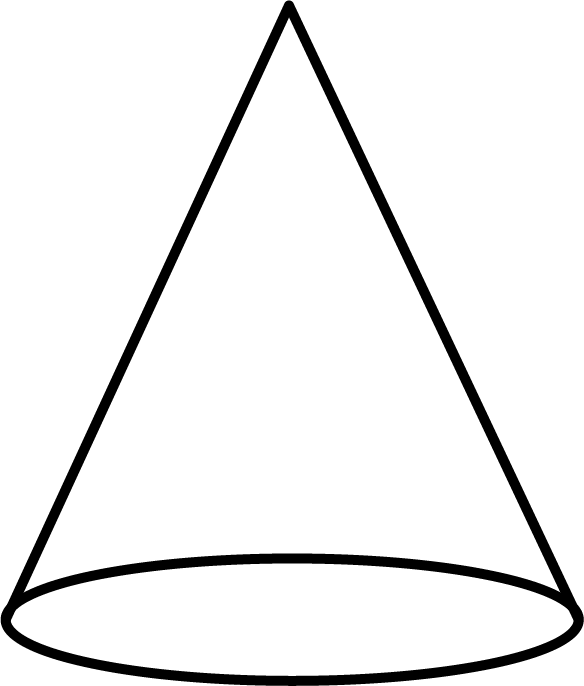(ii)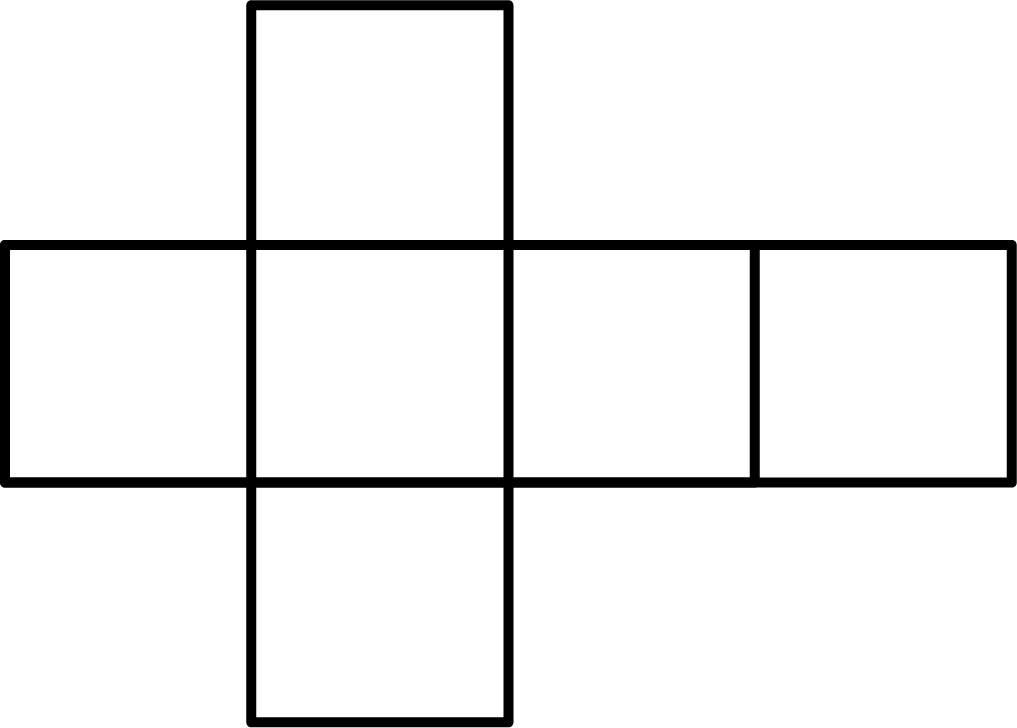(c)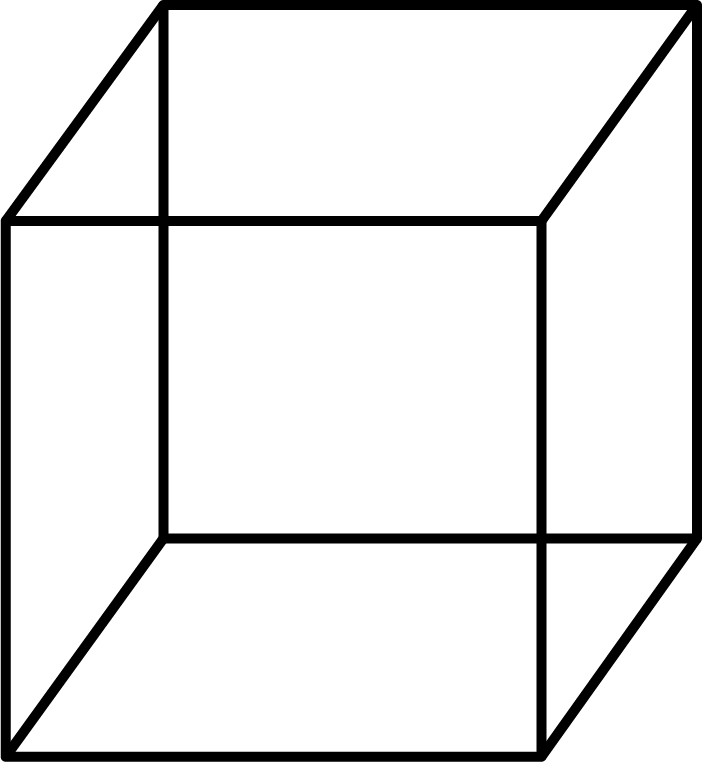(iii)(d)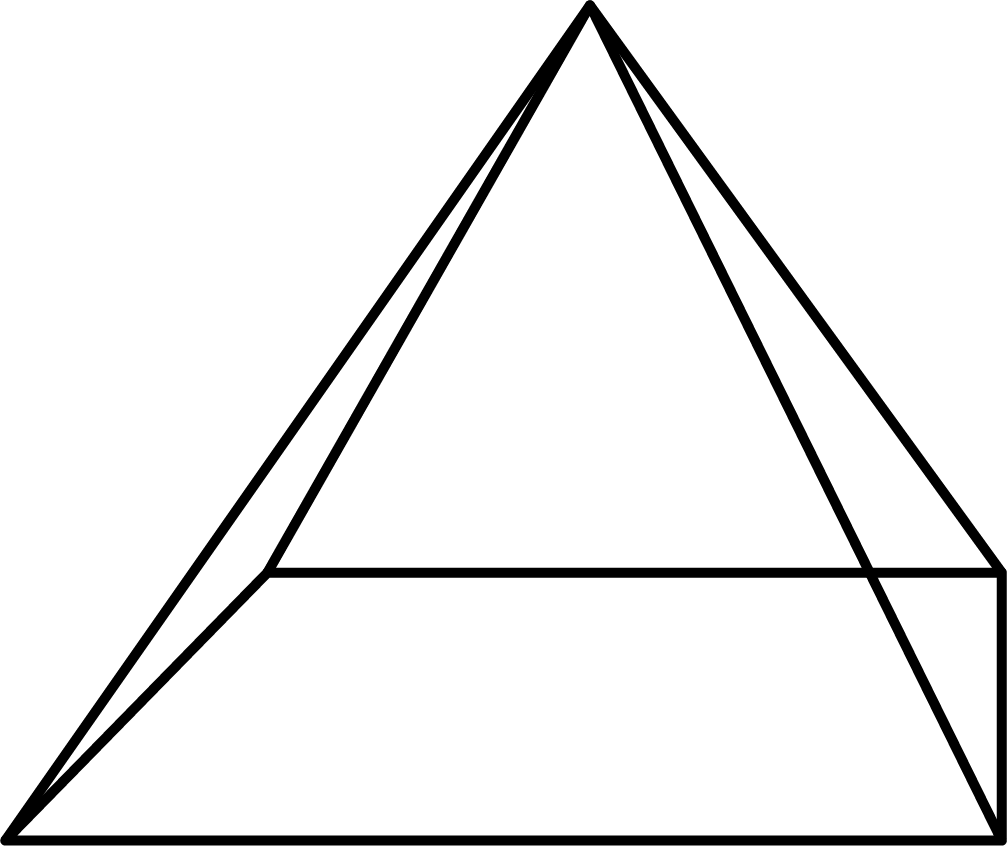(iv)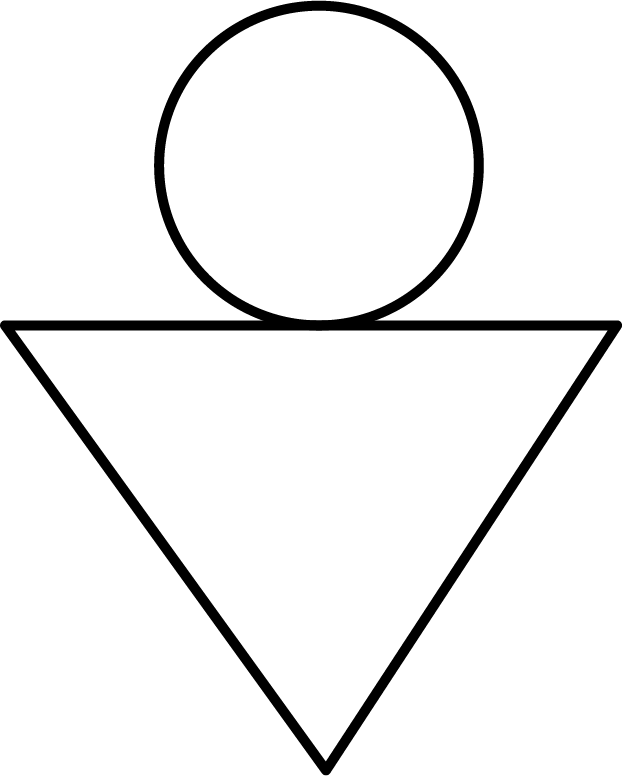Ans:

$(a) - \left( {iii} \right)$

$(b) - \left( {iv} \right)$

$(c) - \left( {ii} \right)$

$(d) - \left( i \right)$

25. What cross sections are obtained when

(i) vertical cut

(ii) horizontal cut

(a) An ice cream cone

(b) A die

(c) A round apple

(d) A circular pipe are done with

Ans:

 S. No Name of the article Figure Vertical cut Horizontal cut (a) An ice cream cone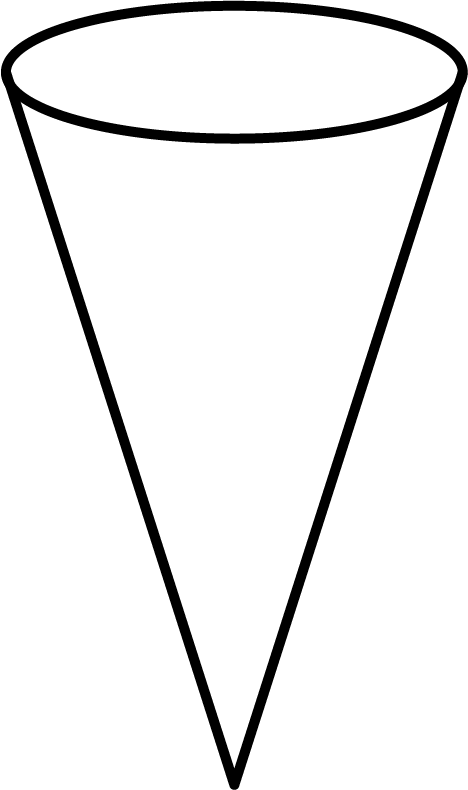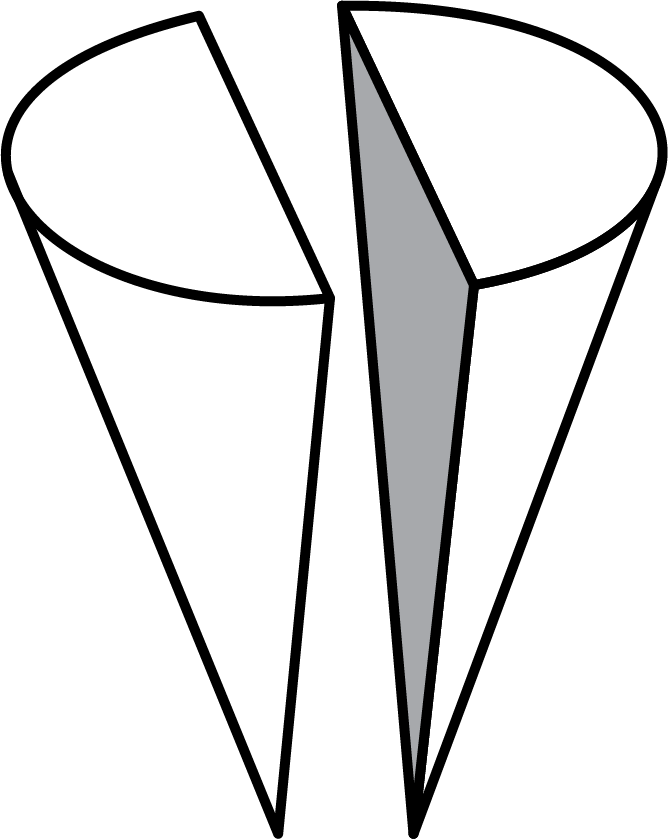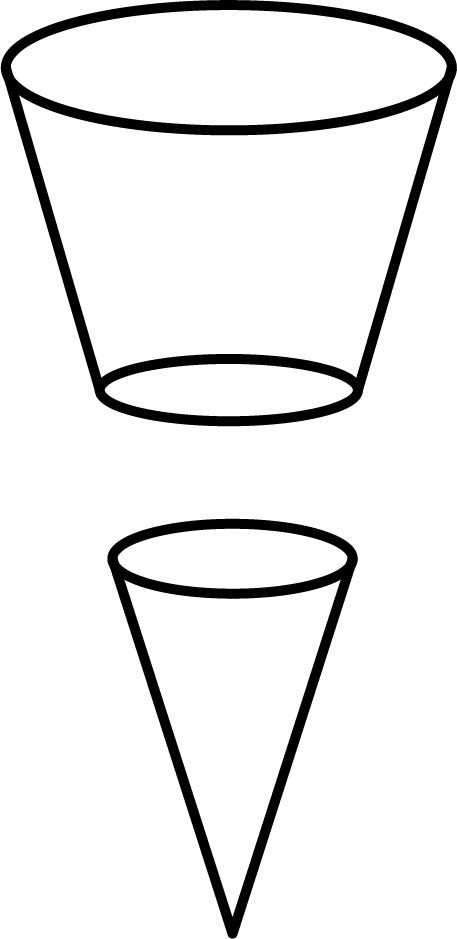(b) A Die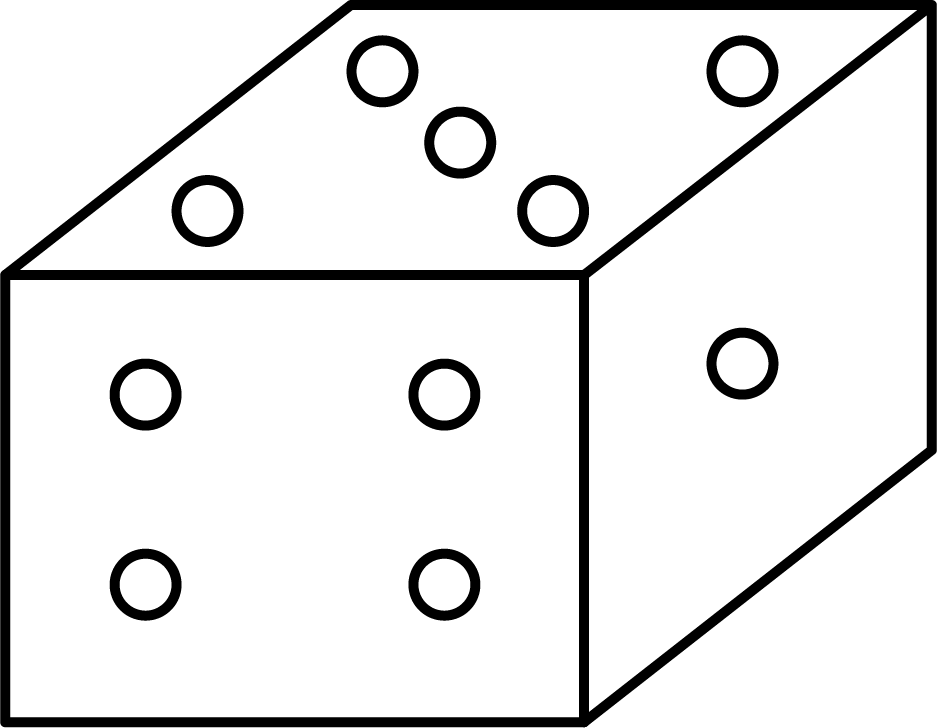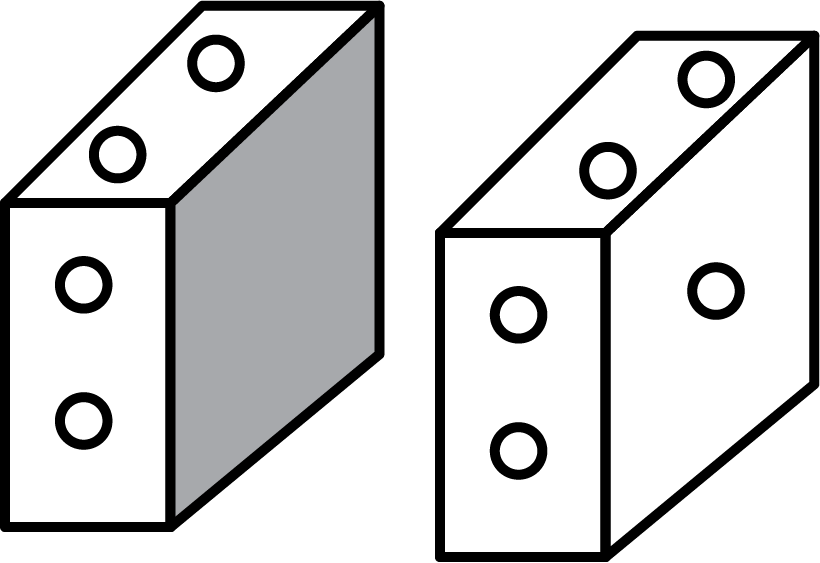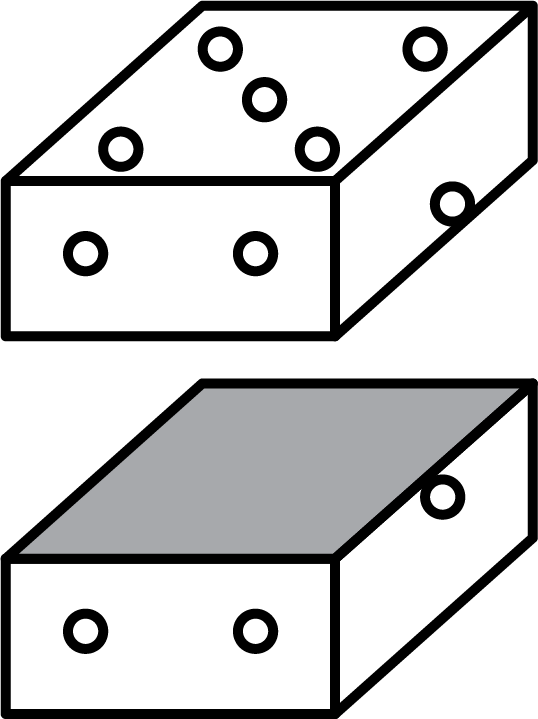(c) A round apple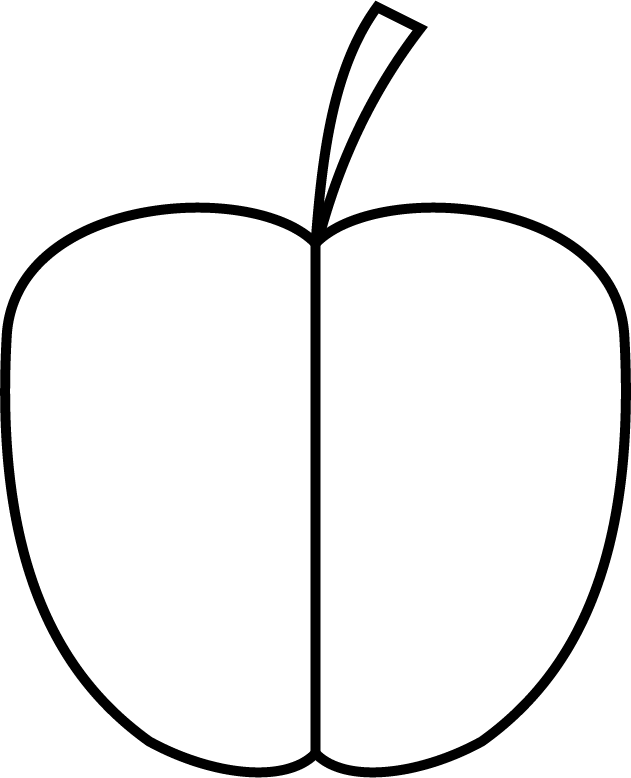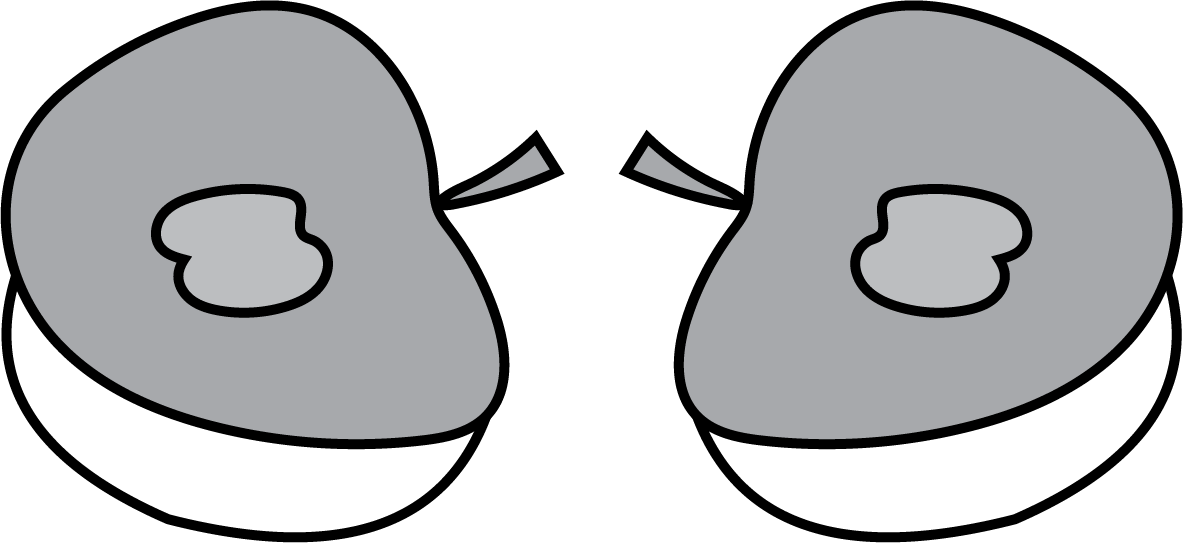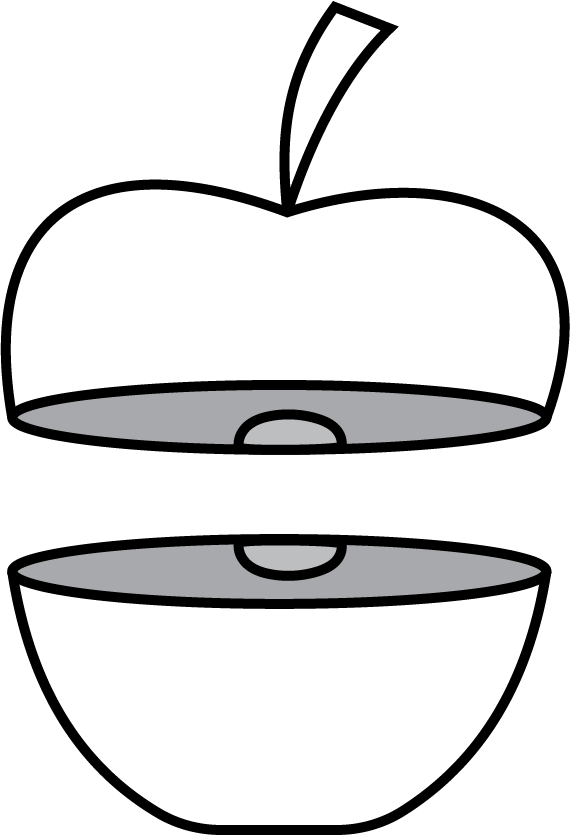(d) A circular pipe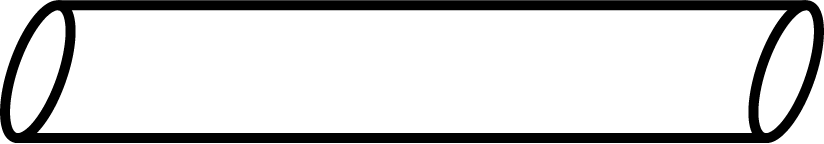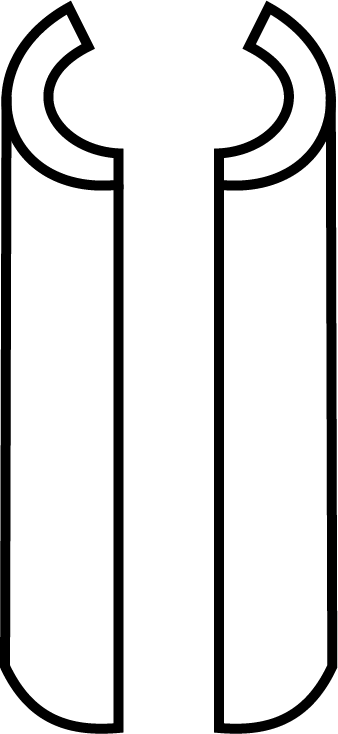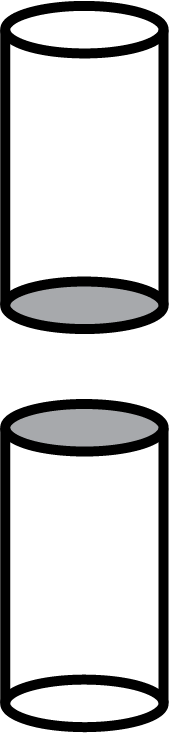## Significance of CBSE Class 8 Maths Chapter 10 Visualising Solid Shapes Important Questions.

The important questions have been formulated by following the latest CBSE Class 8 Maths syllabus to cover all the topics taught in this chapter. It means the students will find problems related to all the mathematical principles taught in this chapter and can practice solving them. Solving these questions will help students understand how to use these concepts skillfully to find accurate answers.

## Advantages of Class 8 Maths Chapter 10 Visualising Solid Shapes Important Questions

• Solving Class 8 Maths Chapter 10 important questions will help you focus on how to use the mathematical concepts of this chapter.

• You can also find out the gaps in your preparation by comparing your answers to the solutions.

• Focus on the stepwise methods to solve these questions to escalate your problem-solving and answering skills.

• Resolve doubts related to these questions with the help of solutions.

## Download CBSE Class 8 Maths Chapter 10 Visualising Solid Shapes Important Questions PDF

Get the free PDF version of these important questions and solutions and complete your study material for this chapter. Practice solving them and take your preparation to the next level.

## FAQs on Important Questions for CBSE Class 8 Maths Chapter 10 - Visualising Solid Shapes

1. Why should I learn solid shapes?

Studying this chapter will enable students to learn more about regular and irregular solids and their features. They can easily identify them later and recall these features.

2. How can I master the problem-solving skills for this chapter?

Focus on solving all the questions of the NCERT exercises first. Then, solve these important questions and compare your answers to the solutions.

3. What is the best way to prepare Class 8 Maths Chapter 10 Visualising Solid Shapes?

Study the theoretical part first. Focus on how they are explained and used to solve the exercise questions by referring to the NCERT solutions. Practice solving the important questions to test your skills. This is how you can prepare this chapter well.

4. Can I use the solutions to revise this chapter?

Following the solutions for these questions will help you recall the methods of solving the important questions and help you revise this chapter.

5. Is it necessary to solve important questions for Class 8 Maths Chapter 10, Visualising Solid Shapes?

The more you practice solving such questions, the better you will score in the exams.